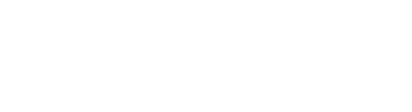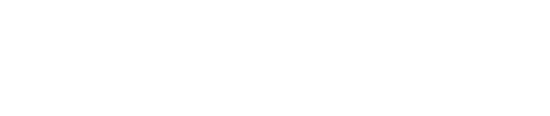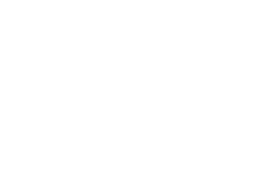Master SAS in 30 days!

A jargon-free, easy-to-learn SAS base course that is tailor-made for students with no prior knowledge of SAS.

# Lesson 5.2: Mean & Standard Deviation

This lesson will explain how to calculate simple statistics such as Mean and Standard Deviation.

ExampleThe codes below compute the mean and standard deviation for each student.

DATA MEAN_AND_SD;

Set Results;

Mean = Mean(ENG, MATH, SCIENCE);
STD = STD (ENG, MATH, SCIENCE);

RUN;DONE! You have learned to compute mean and standard deviation in SAS.

Note:

1. Some other common SAS computing functions are:
- Min (variables, ...): returns minimum result
- Max (variables, ...): returns maximum result
- N (variables, ...): returns number of non-missing result
- NMiss (variables, ...): returns number of missing result
- STDERR (variables, ...): returns standard error
- Var (variables, ...): returns variance
2. Mean and Standard Deviation can also be done using PROC MEANS or PROC UNIVARIATE. These two procedures will be explained in later lessons.

## Master SAS in 30 Days

Article Rating
Subscribe
Notify of